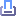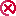霸州市行政审批局关于霸州市全市污水处理设施及配套管网工程全覆盖项目(北部区域)公路建筑控制区内埋设管线、电缆等设施许可[G106(K81+678-K83+750、K83+750-K84+450、K84+450-K88+900、K84+450-K85+465、K85+465-K86+490、K86+490-K86+890、K86+890-K88+400)，G112(K75+361-K74+113、K74+450-K78+330)]的公示 发布时间：2022-12-30

 项目名称 霸州市全市污水处理设施及配套管网工程全覆盖项目(北部区域) 公路建筑控制区内埋设管线、电缆等设施许可[G106(K81+678-K83+750、K83+750-K84+450、K84+450-K88+900、K84+450-K85+465、K85+465-K86+490、K86+490-K86+890、K86+890-K88+400)，G112(K75+361-K74+113、K74+450-K78+330)] 申请单位 中城乡（霸州）水务环保有限公司 施工地点 G106(K81+678-K83+750、K83+750-K84+450、K84+450-K88+900、K84+450-K85+465、K85+465-K86+490、K86+490-K86+890、K86+890-K88+400)，G112(K75+361-K74+113、K74+450-K78+330) 批准日期 2022年12月30日打印此页关闭窗口
 @2003-2018 版权所有：河北省霸州市人民政府　霸州市人民政府办公室承办　运维：霸州市政府信息中心　 网站标识码：1310810001　冀ICP备14014833号-1　冀公网安备 13108102000364号